# Python Program to Convert Binary to Octal

In this article, we've created some programs in Python, to convert any binary number entered by user at run-time to its equivalent octal value. Here are the list of programs:

• Binary to Octal with User-defined Code
• Binary to Octal using int() and oct() Methods
• Shortest Python Code for Binary to Octal Conversion

Note - Before starting these programs, if you're not aware about steps used for the conversion, then refer to Binary to Octal Conversion Methods, Steps, Formula to get every required things.

## Binary to Octal with User-defined Code

To convert binary to octal number in Python, you have to ask from user to enter a number in binary number system to convert that number into octal number system as shown in the program given below.

The question is, write a Python program to convert binary to octal using while loop. Here is its answer:

```print("Enter the Binary Number: ")
binarynum = int(input())

octaldigit = 0
octalnum = []
i = 0
mul = 1
chk = 1
while binarynum!=0:
rem = binarynum % 10
octaldigit = octaldigit + (rem * mul)
if chk%3==0:
octalnum.insert(i, octaldigit)
mul = 1
octaldigit = 0
chk = 1
i = i+1
else:
mul = mul*2
chk = chk+1
binarynum = int(binarynum / 10)

if chk!=1:
octalnum.insert(i, octaldigit)

print("\nEquivalent Octal Value = ", end="")
while i>=0:
print(str(octalnum[i]), end="")
i = i-1
print()```

Here is its initial output: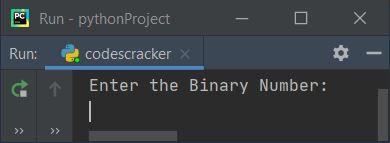Now supply any binary number as input say 11101 and press `ENTER` key to convert it into its equivalent octal value, then print the octal value on output as shown in the snapshot given below: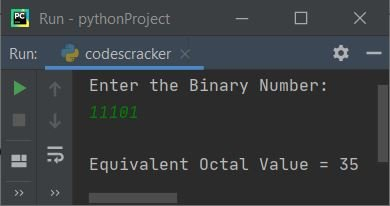The insert() method is used to insert an element to the list. That is, the following statement:

`octalnum.insert(i, octaldigit)`

states that the value of octaldigit gets initialized to octalnum[i].

The dry run of above program with user input 11101 goes like:

• Initial values, binarynum=11101 (entered by user), octaldigit=0, i=0, mul=1, chk=1
• The condition (of while loop) binarynum!=0 or 11101!=0 evaluates to be true, therefore program flow goes inside the loop
• Inside the loop, the first statement, that is
`rem = binarynum % 10`
gets executed
• binarynum%10 or 11101%10 or 1 (last digit of 11101) gets initialized to rem. So rem=1
• octaldigit + (rem*mul) or 0 + (1*1) or 1 gets initialized to octaldigit. So octaldigit=1
• Now the condition (of if block) chk%3==0 or 1%3==0 evaluates to be false, therefore program does not goes to its body, rather it goes to its else's counterpart and mul*2 or 1*2 or 2 gets initialized to mul. So mul=2
• chk+1 or 1+1 or 2 gets initialized to chk. So chk=2
• int(binarynum/10) or int(11101/10) or 1110 gets initialized to binarynum. So binarynum=1110
• The condition of while loop again gets evaluated with new value of binarynum
• That is, the condition binarynum!=0 or 1110!=0 evaluates to be true, therefore program flow again goes inside the loop. This process continues until the condition evaluates to be false
• In this way, the equivalent octal value of value stored in binarynum gets stored in octalnum list after exiting from the loop

The following condition:

`if chk%3==0:`

is applied to check for three-three pair of binary digits. And the following block of code:

```if chk!=1:
octalnum.insert(i, octaldigit)```

is used to insert the value of octaldigit to octalnum[i], only if the value of chk is not equal to 1. The value of chk does not equal to 1 after exiting from the while loop indicates that the program flow does not goes inside the if's body before exiting from the loop.

Now print the value of octalnum list one by one starting from its last index. The end= is used to skip printing of an automatic newline using print()

#### Modified Version of Previous Program

This is the modified version of previous program. This program uses string instead of list, which is a better way in Python to convert any binary number to its equivalent octal value.

```print("Enter the Binary Number: ", end="")
bnum = int(input())

odig = 0
mul = chk = 1
onum = ""
while bnum!=0:
rem = bnum % 10
odig = odig + (rem * mul)
if chk%3==0:
onum = onum + str(odig)
mul = chk = 1
odig = 0
else:
mul = mul*2
chk = chk+1
bnum = int(bnum / 10)

if chk!=1:
onum = onum + str(odig)

print("\nEquivalent Octal Value = ", onum[::-1])```

Here is its sample run with user input, 11000111011 as binary number: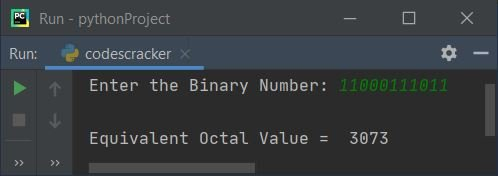Note - The str() method is used to convert any type of value to a string type value.

## Binary to Octal using int() and oct()

This program uses int() and oct() methods to convert binary to octal. Using input() to receive any input from user, treats the input as a string type value by default.

Therefore using int() with 2 as its second argument, converts the string to an integer type value with base two, that is, the input gets converted into a binary number. And oct() converts the value of onum to its equivalent octal value.

```print("Enter a Binary Number: ", end="")
bnum = input()

onum = int(bnum, 2)
onum = oct(onum)

print("\nEquivalent Octal Value = ", onum)```

Here is its sample run with user input, 10111: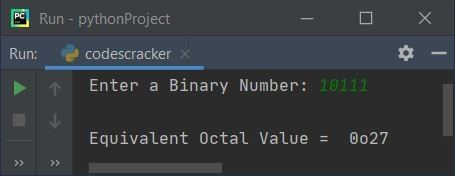Note - To print only 27, that is, if you want to skip first two characters from octal number, then replace the following statement:

`print("\nEquivalent Octal Value = ", onum)`

with the statement given below:

`print("\nEquivalent Octal Value = ", onum[2:])`

Now the output with same user input looks like: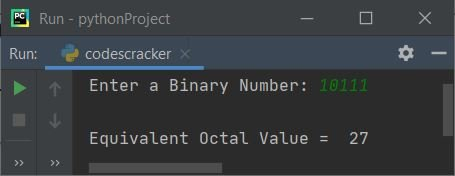### Shortest Python Code for Binary to Octal Conversion

This is the shortest Python code to convert binary to octal. [2:] is used to print elements of bnum starting from its second index.

```bnum = input()
print(oct(int(bnum, 2))[2:])```

Here is its sample run with user input, 1100110: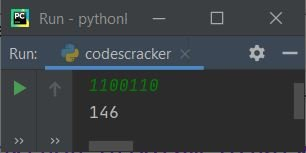#### Same Program in Other Languages

Python Online Test

« Previous Program Next Program »